2.5. Petrosian index and magnitude

The Petrosian (1976, his Equation 7) function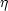(R) is given as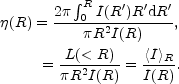(26) (27)

It is the average intensity within some projected radius R divided by the intensity at that radius. The logarithmic expression is written as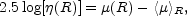(28)

and is shown in Figure (6) for a range of profile shapes n.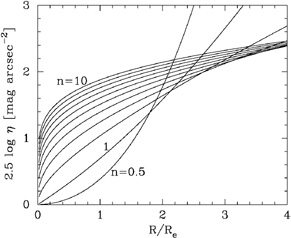Figure 6. The logarithm of the Petrosian function(R) (Equation 28) is shown as a function of normalised radius R / Re for Sérsic profiles having n = 0.5, 1, 2, 3,... 10.

It turns out the Petrosian function is equal to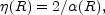(29)

where(R) is given in Equation (24; Djorgovski & Spinrad 1981; Djorgovski, Spinrad & Marr 1984; Sandage & Perelmuter 1990, their Section IIa; Kjærgaard, Jorgensen, & Moles 1993). Thus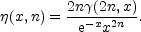(30)

The flux within twice the radius RP when 1 /(RP) = 0.2 is often used to estimate an object's flux (e.g., Bershady, Jangren, & Conselice 2000; Blanton et al. 2001), as is the flux within 3RP when 1 /(RP) = 0.5 (e.g., Conselice, Gallagher, & Wyse 2002; Conselice et al. 2003). How well this works of course depends on the shape of the light-profile, and Figure (7) shows these approximations to the total luminosity as a function of the Sérsic index n. In the case of 2RP when 1 /(RP) = 0.2, one can see that profiles with n = 10 will have their luminosities under-estimated by 44.7% and those with n = 4 by only 17.1%. The situation is considerably worse when using 3RP and 1 /(RP) = 0.5. A prescription to correct for the missing light, beyond one's chosen aperture, is detailed in Graham et al. (2005).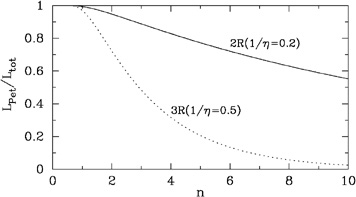Figure 7. Flux ratio, as a function of light-profile shape n, between the total luminosity Ltot and the Petrosian luminosity LPet inside (i) twice the radius RP where 1 /(RP) = 0.2 (solid curve) and (ii) thrice the radius RP where 1 /(RP) = 0.5 (dotted curve).